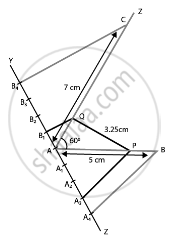# Two line segments AB and AC include an angle of 60° where AB = 5 cm and AC = 7 cm. Locate points P and Q on AB and AC, respectively such that AP = 34 AB and AQ = 14 AC. Join P and Q and measure the - Mathematics

Diagram
Sum

Two line segments AB and AC include an angle of 60° where AB = 5 cm and AC = 7 cm. Locate points P and Q on AB and AC, respectively such that AP = 3/4 AB and AQ = 1/4 AC. Join P and Q and measure the length PQ.

#### Solution

Steps of construction:

1. Draw a line segment AB = 5 cm.2. Draw ∠BAZ = 60°.

3. With centre A and radius 7 cm, draw an arc cutting the line AZ at C.

4. Draw a ray AX, making an acute ∠BAX.

5. Divide AX into four equal parts, namely AA= A1A2 = A2A3 = A3A4.

6. Join A4B.

7. Draw A3P || A4B meeting AB at P.

8. Hence, we obtain, P is the point on AB such that AP = 3/4 AB.

9. Next, draw a ray AY, such that it makes an acute ∠CAY.

10. Divide AY into four parts, namely AB1 = B1B= B2B3 = B3B4.

11. Join B4C.

12. Draw B1Q || B4C meeting AC at Q. We get, Q is the point on AC such that AQ = 1/4 AC.

13. Join PQ and measure it.

14. PQ = 3.25 cm

Concept: Division of a Line Segment
Is there an error in this question or solution?

#### APPEARS IN

NCERT Mathematics Exemplar Class 10
Chapter 10 Construction
Exercise 10.4 | Q 1 | Page 117
Share